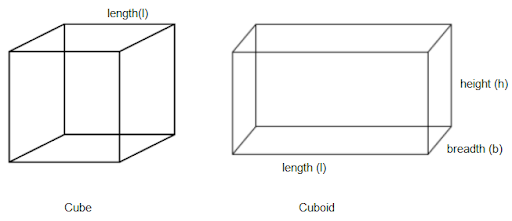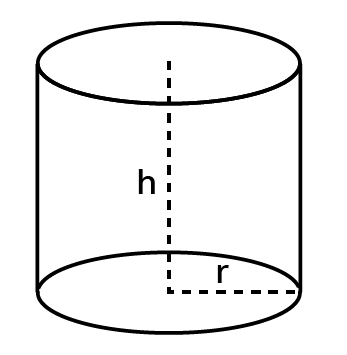Courses
Courses for Kids
Free study material
Offline Centres
More

# Mensuration Class 8 Notes CBSE Maths Chapter 11 (Free PDF Download)Last updated date: 01st Dec 2023
Total views: 592.8k
Views today: 15.92k

## Revision Notes for CBSE Class 8 Maths Chapter 11 - Free PDF Download

CBSE Mensuration Class 8 Notes Mensuration offered by Vedantu are available in PDF format to help students with their exam preparation. These revision notes Maths Class 8 Chapter 11 is written as per the latest CBSE syllabus to help you to cover all the relevant topics and formulas. Vedantu strives to provide Revision Notes Maths Class 8 Chapter 11 to help you to revise the entire chapter within a short period. Our Mensuration Class 8 Notes are available in an easily downloadable PDF format that will enhance your preparation for any assignments or exams. You can make use of Maths Class 8 Mensuration notes to clear your doubts and get a  deeper understanding of the chapter.

You can also register Online for Class 8 Science tuition on Vedantu.com to score more marks in CBSE board examination. Vedantu is a platform that provides free CBSE Solutions (NCERT) and other study materials for students. Maths Students who are looking for the better solutions ,they can download Class 8 Maths NCERT Solutions to help you to revise complete syllabus and score more marks in your examinations.

Also, check CBSE Class 8 Maths revision notes for All chapters:

## Access Class 8 Math Chapter 11 - Mensuration Notes

• Perimeter: A simple closed figure's perimeter is the length of its boundary.

Rectangle$=2\left( l+b \right)$

Square$=4a$

Parallelogram$=2$(sum of adjacent sides)

• Area: The size of a territory encompassed in a simple closed figure is referred to as its area.

• The area of a trapezium is equal to half of the sum of the parallel sides' lengths divided by the perpendicular distance between them.

\begin{align} & \text{Area of a rhombus = half the product of its diagonals}\text{. }\!\!~\!\!\text{ } \\& \text{Triangle = }\dfrac{1}{2}\text{ }\!\!~\!\!\text{ }\times \text{ base }\times \text{ height }\!\!~\!\!\text{ } \\ \end{align}

• Diagonal of:

Rectangle$=\sqrt{{{l}^{2}}+{{b}^{2}}}$

Square$=\sqrt{2a}$

• A solid's surface area is equal to the sum of its face areas.

Surface area of cuboid $\text{=2}\left( lb+bh+lh \right)$

Surface area of cube $=6{{l}^{2}}$Surface area of a cylinder $=2\pi r\left( r+h \right)$The volume of a solid is the amount of space it takes up.

Volume of

a cuboid $=l\times b\times h$

a cube $={{l}^{3}}$

a cylinder $=\pi {{r}^{2}}h$

Few basic measurements value are as follows:

$\left( i \right)1c{{m}^{3}}=1mL$

$\left( ii \right)1L=1000c{{m}^{3}}$

$\left( iii \right)1{{m}^{3}}=1000000c{{m}^{3}}$

$=1000L$

Vedantu is an online platform that initiates to provide free Revision Notes Maths Class 8 Chapter 11 and other study material for students. These can be easily downloaded and students can easily use it as future reference. Class 8 Chapter 11 will become quite easy for you to study if you refer to Mensuration Class 8 Chapter 11 NCERT Solutions along with Mensuration Class 8 Notes provided by Vedantu.

Maths Class 8 Mensuration Notes offered by Vedantu is important for aspiring students who are aiming to score top marks in their examination. This revision notes Class 8 Maths Chapter 11 will help you in preparing for your upcoming Class 8 examination as well as other competitive exams like Olympiad. Opt for Maths Class 8 Mensuration notes online to learn about various shortcut techniques that help you to solve numerical questions in a step by step manner.

Download revision notes Class 8 Maths Chapter 11 free PDF just with a single click on the PDF link given below and gain access anytime and anywhere at your convenience. Mastering the revision notes will help you get an idea about the type of questions and time required to solve the questions given in Chapter 11 Mensuration. This will enable you to improve your performance and understanding of the chapter.

### A Quick Glimpse of  Class 8 Chapter 11 Mensuration

This chapter 11 titled Mensuration introduces you with different geometrical figures and their parameters like perimeter, area, surface area, length, volume, lateral surface area, etc. In your previous classes, you must have learned that for a close figure, the perimeter is defined as the distance around its boundary and the area of the closed figure is the region covered by it. You must have also learned to calculate the area and perimeter of different plane figures such as circles, triangles, rectangles, etc. You must also be aware of the formulas used to calculate the area of pathways or borders in a rectangular shape.

In this chapter Mensuration, you will learn to find the perimeter and area of other closed figures such as Quadrilateral. You will also learn to find the surface area and volume of solids such as a cube, cuboid, and cylinder.

Students should have clarity on the important formulas and concepts of Mensuration so that they do not face any issues in solving the numerical questions related to Mensuration in their CBSE Class 8 exams.

### Topics and Subtopics Covered in Chapter 11 Mensuration

Here, you can find the list of the topics and subtopics covered in Chapter 11 Mensuration.

(Table will be updated soon)

### Mensuration Important Formulas

Some important Mensuration formulas are discussed below:

• Area of Trapezium

½ of the sum of the length of parallel sides * Perpendicular distance between them.

• Surface Area of Cuboid  - 2 (LB + BH + HL)  or  2 (Length * Breadth + Breadth * Height + Length * Height )

• Surface Area of Cube - 6 * side²

• Volume of Cuboid -  LBH or (Length  * Breadth * Height)

• Volume  of Cube - Side³

• Volume of Cylinder - πr²h

In the above formula

H - Height of cylinder

To get a detailed view of this chapter, download our latest Class 8 Maths revision notes Chapter 11 free PDF. This free PDF will help you to understand all the important concepts related to Mensuration and solve all your doubts regarding the chapter.

### Key Highlights of CBSE Class 8 Maths Revision Notes Chapter 11

Some of the key highlights of CBSE Class 8 Maths revision notes Chapter 11 are discussed below:

• The students can easily understand, practice, and revise all the important concepts of chapter 11 by referring to these revision notes.

• The important topics covered in the revision notes are defined in a crisp and precise manner.

• It saves your lot of valuable time by understanding all the important concepts and formulas of the chapter given in a proper sequence in Maths Class 8 Mensuration notes.

• These Class 8 Maths notes of Mensuration provided by Vedantu include several important tips useful for solving Mensuration numerical questions effectively.

• These notes are written by subject experts at Vedantu in a neat and well- structured manner that makes it attractive and simpler for students to learn.

## FAQs on Mensuration Class 8 Notes CBSE Maths Chapter 11 (Free PDF Download)

1. What is the definition of Mensuration according to Class 8?

Ans: Measuring the area and perimeter of different shapes is known as mensuration. The shapes include triangle, trapezium, rectangle, square, etc. You can find their area by using the required formula. The notes are free of cost. The solutions are available on the Vedantu Mobile app as well. Some example questions are as follows:

1. Find the area of a square whose side is 30 m.

Answer: Formula of the area of square= (Side)^2

Sise as given in question = 30 m.

Thus, the area of the square is = (30)2 = 900 m^2.

2. Find the perimeter of a triangle with two equal sides of 5 cm and one side of 10 cm.

Answer: The formula of the triangle is :

Triangle of given parameter= sum all the three sides of the triangle

= (5 + 5 + 10)cm.

= 20 cm.

2. A flooring tile has the shape of a parallelogram whose base is 24 cm and the corresponding height is 10 cm. How many such tiles are required to cover a floor of area 1080 m2? (If required you can split the tiles in whatever way you want to fill up the corners)

Ans:

The base of the floor title is given as = 24 cm = 0.24 m

Height of the corresponding floor title= 10 cm = 0.10 m

Area of the floor title is= Base×Altitude

= 0.24×0.10

= 0.024

Thus the area of the flooring title is =0.024m2

Number of tiles that will be required for covering the floor= Area of floor/Area of one tile = 1080/0.024

= 45000 tiles

3. Find the area of a rhombus whose side is 5 cm and whose altitude is 4.8 cm. If one of the diagonals is 8 cm long, find the length of the other diagonal.

Ans: The formula of rhombus and parallelogram are similar,

The formula we get of a rhombus is = Base×Altitude

Placing the values, we get,

Area of rhombus = 6×4 = 24

The area of a rhombus is 24 cm2

The formula of rhombus = (½)×d1d2

Through the substitution formula, we get

24 = (½)×8×d2

d2 = 6

So the diagonal length is 6 cm

4. The floor of a building consists of 3000 rhombus-shaped tiles and each of its diagonals is 45 cm and 30 cm in length. Find the total cost of polishing the floor, if the cost per m^2 is Rs. 4.

Ans:

Given length of the diagonal is, d1 = 45 cm and d2= 30 cm

We get the Area of one tile after putting the value = (½)d1d2

= (½)×45×30 = 675

Hence it isis 675 cm2

Area of 3000 tiles is

= 675×3000 = 2025000 cm2

= 2025000/10000

= 202.50 m2

cost for polishing the floor per sq. meter = 4

Cost we get per 202.50 sq. meter = 4×202.50 = 810

Total cost is= 810

5.What all things students will learn from Chapter 11 of Class 8 Maths?

Ans: The surface area and volume of these figures are thoroughly studied by the children. This chapter is vital not just for students in class 8, but also for students in higher grades. As a result, children must establish a solid foundation in this subject.

Chapter 11 of the NCERT answers for Class 8 maths is knowledge-packed. Aside from that, pupils are taught how to use visualization to their advantage. If students are asked to find the surface area of a cube, for example, they must mentally visualize the shape and comprehend its components to answer problems based on it.